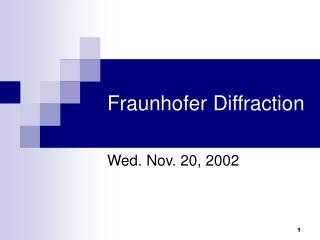DownloadDownload PresentationFraunhofer Diffraction

# Fraunhofer Diffraction

Télécharger la présentation## Fraunhofer Diffraction

- - - - - - - - - - - - - - - - - - - - - - - - - - - E N D - - - - - - - - - - - - - - - - - - - - - - - - - - -
##### Presentation Transcript

1. Fraunhofer Diffraction Wed. Nov. 20, 2002

2. Kirchoff integral theorem This gives the value of disturbance at P in terms of values on surface  enclosing P. It represents the basic equation of scalar diffraction theory

3. Geometry of single slit Have infinite screen with aperture A Let the hemisphere (radius R) and screen with aperture comprise the surface () enclosing P.  P S r r’ ’  Radiation from source, S, arrives at aperture with amplitude Since R  E=0 on . R  Also, E = 0 on side of screen facing V.

4. Fresnel-Kirchoff Formula • Thus E=0 everywhere on surface except the portion that is the aperture. Thus from (6)

5. Fresnel-Kirchoff Formula • Now assume r, r’ >>  ; then k/r >> 1/r2 • Then the second term in (7) drops out and we are left with, Fresnel Kirchoff diffraction formula

6. Obliquity factor • Since we usually have ’ = - or n.r’=-1, the obliquity factor F() = ½ [1+cos ] • Also in most applications we will also assume that cos   1 ; and F() = 1 • For now however, keep F()

7. Huygen’s principle • Amplitude at aperture due to source S is, • Now suppose each element of area dA gives rise to a spherical wavelet with amplitude dE = EAdA • Then at P, • Then equation (6) says that the total disturbance at P is just proportional to the sum of all the wavelets weighted by the obliquity factor F() • This is just a mathematical statement of Huygen’s principle.

8. In Fraunhofer diffraction, both incident and diffracted waves may be considered to be plane (i.e. both S and P are a large distance away) If either S or P are close enough that wavefront curvature is not negligible, then we have Fresnel diffraction Fraunhofer vs. Fresnel diffraction S P Hecht 10.2 Hecht 10.3

9. Fraunhofer vs. Fresnel Diffraction  ’  r’ r h’ h d’ S P d

10. Fraunhofer Vs. Fresnel Diffraction Now calculate variation in (r+r’) in going from one side of aperture to the other. Call it 

11. Fraunhofer diffraction limit sin’ sin • Now, first term = path difference for plane waves ’  sin’≈ h’/d’ sin ≈ h/d sin’ + sin =  ( h’/d + h/d ) Second term = measure of curvature of wavefront Fraunhofer Diffraction 

12. Fraunhofer diffraction limit • If aperture is a square -  X  • The same relation holds in azimuthal plane and 2 ~ measure of the area of the aperture • Then we have the Fraunhofer diffraction if, Fraunhofer or far field limit

13. Fraunhofer, Fresnel limits • The near field, or Fresnel, limit is • See 10.1.2 of text

14. Fraunhofer diffraction • Typical arrangement (or use laser as a source of plane waves) • Plane waves in, plane waves out screen S  f1 f2

15. Fraunhofer diffraction • Obliquity factor Assume S on axis, so Assume  small ( < 30o), so • Assume uniform illumination over aperture r’ >>  so is constant over the aperture • Dimensions of aperture << r r will not vary much in denominator for calculation of amplitude at any point P consider r = constant in denominator

16. Fraunhofer diffraction • Then the magnitude of the electric field at P is,

17. Single slit Fraunhofer diffraction P y = b  r dy ro y r = ro - ysin dA = L dy where L   ( very long slit)

18. Single slit Fraunhofer diffraction Fraunhofer single slit diffraction pattern